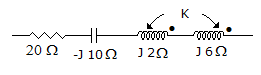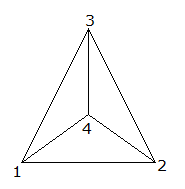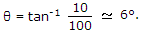# Electronics and Communication Engineering - Networks Analysis and Synthesis

31.

In the series circuit shown in figure, for series resonance, the value of the coupling coefficient, K will beA. 0.25 B. 0.5 C. 0.1 D. 1

Explanation:

Mutual inductance wm = ωk L1L2m = k. 2. 8j2 = 4k. j

Z = 20 - j10 + j2 + j6 + 2.4kj - 2j + 8kj = 0 at resonance-2 = -8k

k = 1/40.25 .

32.

The degree of the nodes 1, 2, 3, 4 isA. 2 B. 3 C. 2 for 1, 2, 3, and 3 for 4 D. all 3

Explanation:

Degree of node is the no.of branch incident to it.

33.

In an RC series circuit R = 100 Ω and XC = 10 Ω. In this circuit

 A. the current and voltage are in phase B. the current leads the voltage by about 6° C. the current leads the voltage by about 84° D. the current lags the voltage by about 6°

Explanation:

In RC circuit the current leads the voltage.34.

The impedance of an RC series circuit is 12 Ω at f= 50 Hz. At f= 200 Hz, the impedance will be

 A. more than 12 B. less than 3 C. more than 3 Ω but less than 12 Ω D. more than 12 Ω but less than 24 Ω

Explanation:

Z = R2 + XC2. When f is made four times, XC becomes one-fourth but R remains the same.

35.

A current is flowing through a conductor with non-uniform area of cross-section. Then

 A. current will be different at different cross-sections. B. current will be the same at all the cross-sections. C. current will be different but current density will be same at all the cross-sections D. current will be the same but current density will be different at different cross-sections.

Explanation:

Current density = current/area of cross-section. Same current throughout the conductor.

Since, area of cross-section is non-uniform, current density will be different at different cross-sections.